9.3 Feature Scaling via Standard Normalization*

* The following is part of an early draft of the second edition of Machine Learning Refined. The published text (with revised material) is now available on Amazon as well as other major book retailers. Instructors may request an examination copy from Cambridge University Press.

In this Section and the following Section we two fundamental methods of input normalization - also called feature scaling. While this sort of feature engineering step provides several benefits to the learning process that we will see throughout this Chapter, here we focus on how it substantially improves learning speed when using first order optimization algorithms. As such this 'optimization trick' proves quite useful in our current context of linear machine learning, and will prove especially valuable when we deal with nonlinear learning in the future - like e.g., deep networks - where training via first order methods is all but essential.

In this Section we first explore the benefit of our first feature scaling technique: standard normalization. We do this by exploring a number of simple supervised learning examples.

In :

## Standard normalization of regression datam¶

Below we load in and show a dataset we wish to perform linear regression on. A quick glance at the data and we know that - if tuned properly - a linear regression will fit to this dataset exceedingly well, as the data appears to be roughly distributed on a line.

In :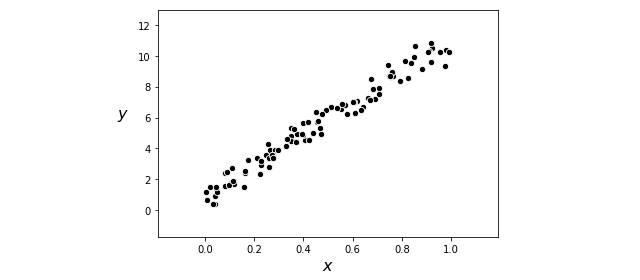Since this is a low dimensional example with only two parameters to learn (the bias and slope of a best fit line) let us take a look at its associated Least Squares cost function. Below we plot its contours in the usual fashion - with darker blue regions corresponding to larger points on the cost surface, and conversely lighter regions indicating lower points on the cost function.

in minimizing the Least Squares cost function using a simple linear regression dataset - which we load in / show below. A quick glance at this dataset and we can tell that linear regression will provide

In :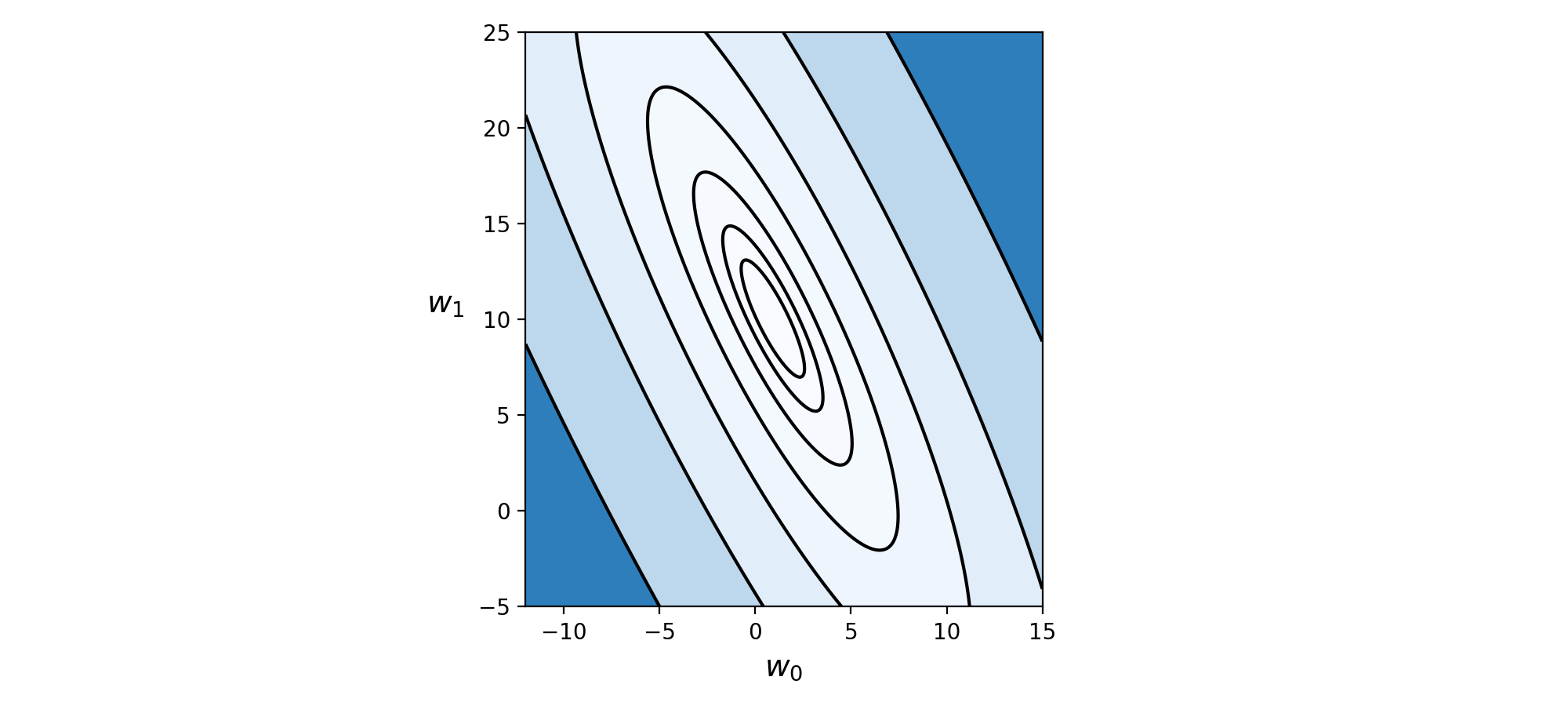Notice how elliptical the contours of this cost function are - these create a long narrow valley along the long axis of the ellipses.

While we can minimize this cost function using a variety of techniques, in this Section we will focus on employing gradient descent. While we can certainly minimize this Least Squares cost using gradient descent, as discussed in Section 3.6 this algorithm progresses quite slowly when applied to minimize a cost functions like the one shown above (with its long narrow valley). So unless we luck out and initialize directly along the short axes of such an elliptical contour-having cost function, gradient descent will need to take a large number of steps to reach an approximate global minimum.

We illustrate this fact by showing a run of $100$ gradient descent steps initialized at the point $\mathbf{w}^0 = \begin{bmatrix} 0 \\ 0 \end{bmatrix}$. Here we use a fixed steplength parameter $\alpha = 10^{-1}$. Here $10^{-1}$ was the largest value of $\alpha$ of the form $10^{-\gamma}$ we found that produced convergence from this initialization (larger values of $\alpha$ caused gradient descent to diverge).

In :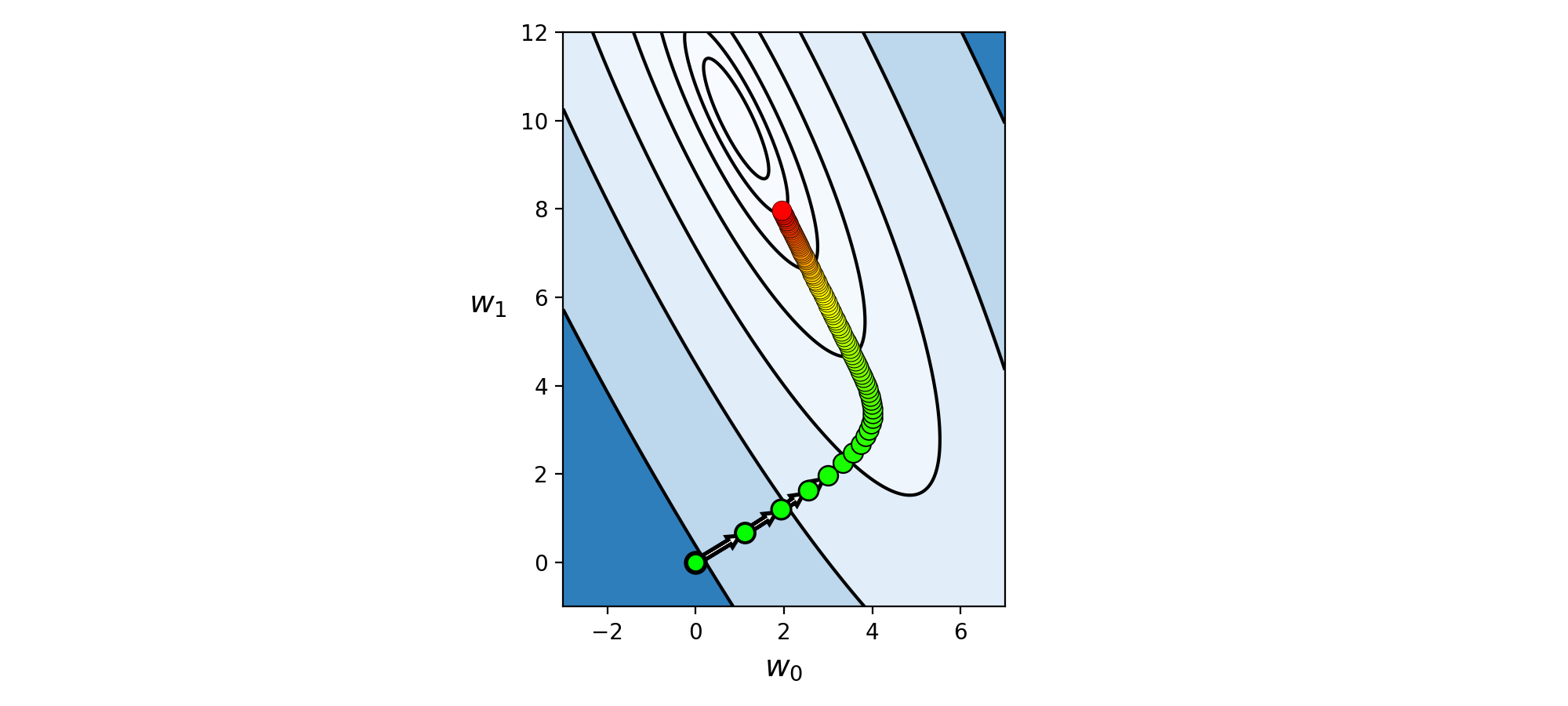Here as usual the steps are colored from green to red as gradient descent begins (green) to when it ends (red). From this perspective we can see that we still have a way to travel to reach the minimum of the cost function. Moreover, gradient descent is naturally slowing down as we approach the global minimum here - so we will need quite a few additional steps to reach an appropriate solution.

Plotting the line given by the final set of weights learned in this run of standard gradient descent - those associated with the final red point plotted on the contour plot above - we can see that the fact that these weights lie so far from the true minimum of the cost function truly affect the line's quality - we get poor fit considering how simple the dataset is.

In :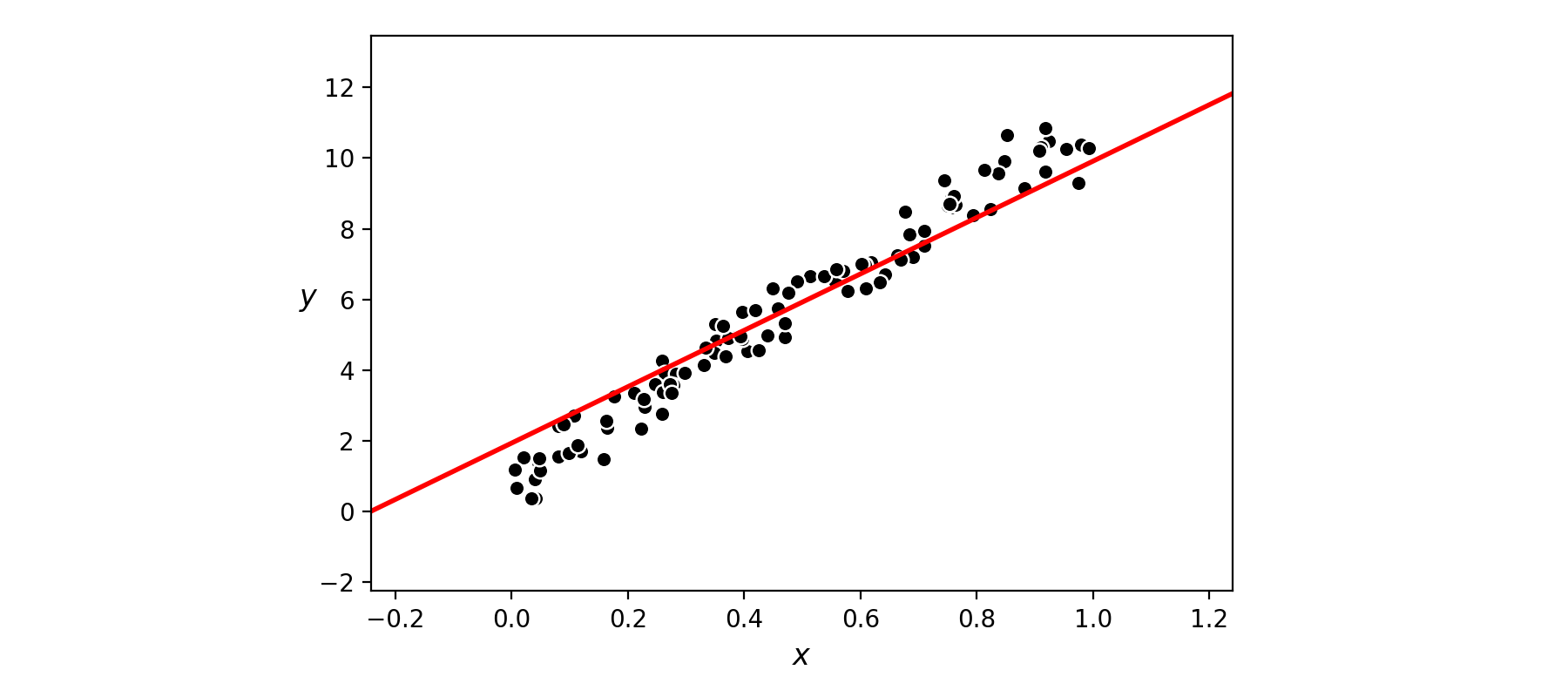We can actually make an extremely simple adjustment of the data ameliorates this issue significantly, altering the shape of our Least Squares cost function so that its contours are much more circular (thus making it considerably easier for gradient descent to find global minima). This simple adjustment is called standard normalization. In particular, we normalize our inputs to have zero mean and unit standard deviation.

What does this look like? Well we just replace each input $x_p$ point with its mean centered unit deviation analog as

\begin{equation} x_p \longleftarrow \frac{x_p - \mu}{\sigma} \end{equation}

where the sample mean of the inputs $\mu$ is defined as

\begin{equation} \mu = \frac{1}{P}\sum_{p=1}^{P}x_p \\ \end{equation}

and the sample standard deviation of the inputs $\sigma$ is defined as

\begin{array} \ \sigma = \sqrt{\frac{1}{P}\sum_{p=1}^{P}\left(x_p - \mu \right)^2}. \end{array}

This simple normalization scheme is often called standard normalization.

As we will see below for the particular dataset we are currently studying, this simple normalization 'trick' has a profound impact on the shape of our cost function. Also note: this normalization scheme is invertible, meaning that after performing it we can always return to our original data by simple re-multiplying a normalized input by the original standard deviation and adding the original mean.

In :
# standard normalization function - returns functions for standard normalizing and reverse standard
# normalizing an input dataset x
def standard_normalizer(x):
# compute the mean and standard deviation of the input
x_means = np.mean(x,axis = 1)[:,np.newaxis]
x_stds = np.std(x,axis = 1)[:,np.newaxis]

# check to make sure thta x_stds > small threshold, for those not
# divide by 1 instead of original standard deviation
ind = np.argwhere(x_stds < 10**(-2))
if len(ind) > 0:
ind = [v for v in ind]

# create standard normalizer function
normalizer = lambda data: (data - x_means)/x_stds

# create inverse standard normalizer
inverse_normalizer = lambda data: data*x_stds + x_means

# return normalizer
return normalizer,inverse_normalizer

In :

Now we form a Least Squares cost function with our normalized input, with the output un-changed.

With our cost function formed we now plot its contours, just as done previously. As you can see - amazingly - the contours of this Least Squares cost (with normalized input) are perfectly circular. No long narrow valleys can ever exist in such a function, and so gradient descent can much more rapidly minimize this cost.

In :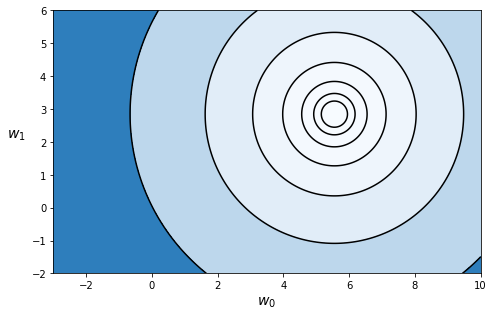Below we show an animation where we form a sequence of Least Squares cost functions using a convex combination of the original and normalized data

\begin{equation} \left(1 - \lambda\right)x_p + \lambda \left( \frac{x_p - \mu}{\sigma} \right) \end{equation}

where $\lambda$ ranges from $0$ (i.e., we use the original input) to $\lambda = 1$ (where we use the normalized versions). Plotting the contour of each Least Squares cost for a $50$ evenly spaced values of $\lambda$ between $0$ and $1$ shows how the original Least Squares cost function is transformed by normalizing the input. You can use the slider below to transition between the contours of the original cost function (when the slider is all the way to the left) and cost function taking in normalized input (when the slider is all the way to the right).

In [ ]:
In :
Out:

In the next Python cell we repeat our previous run of unnormalized gradient descent - beginning at the same initial point $\mathbf{w}^0 = \begin{bmatrix} 0 \\ 0 \end{bmatrix}$, using a steplength parameter $\alpha = 10^{-1}$ (the largest steplength value of the form $10^{-\gamma}$ we could find that caused the algorithm to converge). However here we will only take $20$ steps instead of $100$ as we did before, since we basically reach the minimum after just a few steps now (no more long narrow valleys to struggle through!).

In :

Using only a quarter of the number of descent steps - with precisely the same setup as previously - we get much closer to the cost function minimum!

Let us plot the fit associated with the final set of weights (the final red point above) on our original dataset below. Notice that in order to make this plot we must treat each new input test point on the linear predictor precisely as we treated our original input: i.e., we must subtract off the same mean and divide off the same standard deviation. Thus with our fully tuned parameters $w_0^{\star}$ and $w_1^{\star}$ our linear predictor for any input point $x$ is, instead of $w_0^{\star} + w_1^{\star}x^{\,}$, now

\begin{equation} \text{normalized_predictor}\left(x\right) = w_0^{\star} + w_1^{\star}\left(\frac{x - \mu}{\sigma}\right). \end{equation}

Again - since we normalized the input data we trained on, we must normalize any new input point we shove through our trained linear model.

The final predictor - plotted below in red - is far superior to the one we found previously, where we took $5$ times as many gradient descent steps, prior to normalizing the input data.

In :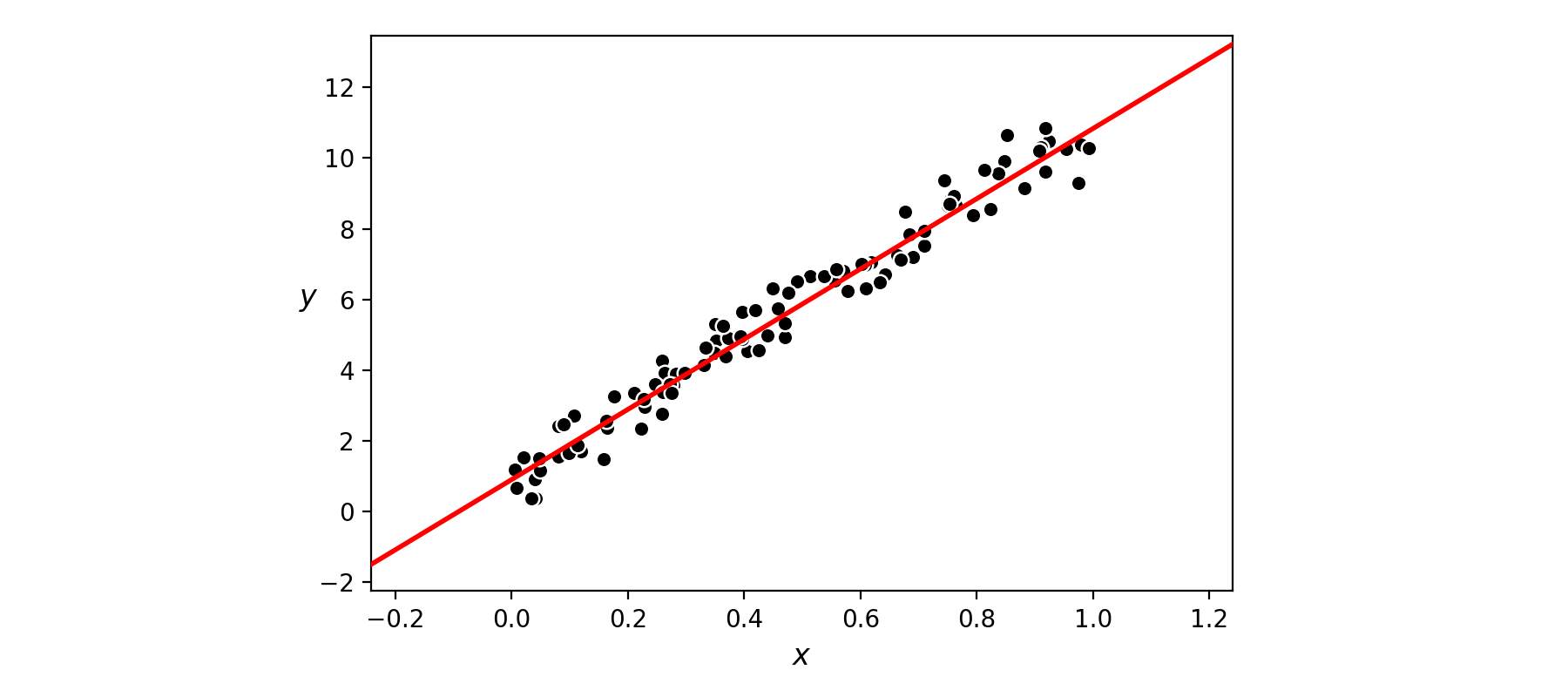Now that we have seen empirical evidence that the standard normalization scheme significantly aids with parameter tuning with single-input linear regression, we explore (by example) how the same normalization scheme likewise helps significantly with the multi-input datasets as well. In the subsections that follow this one we summarize our findings, and provide more rigorous (mathematical) evidence to backup these experimental findings. In particular we will use the $N = 5$ input dimension regression dataset loaded in the below. This dataset consists of a selection of random points taken from a random hyperplane in six dimensions (five inputs, one output), with absolutely no noise whatsoever added to the output.

Lets examine the numerical range of each input dimension / feature. In the next cell we plot a discrete histogram of each input feature.

In :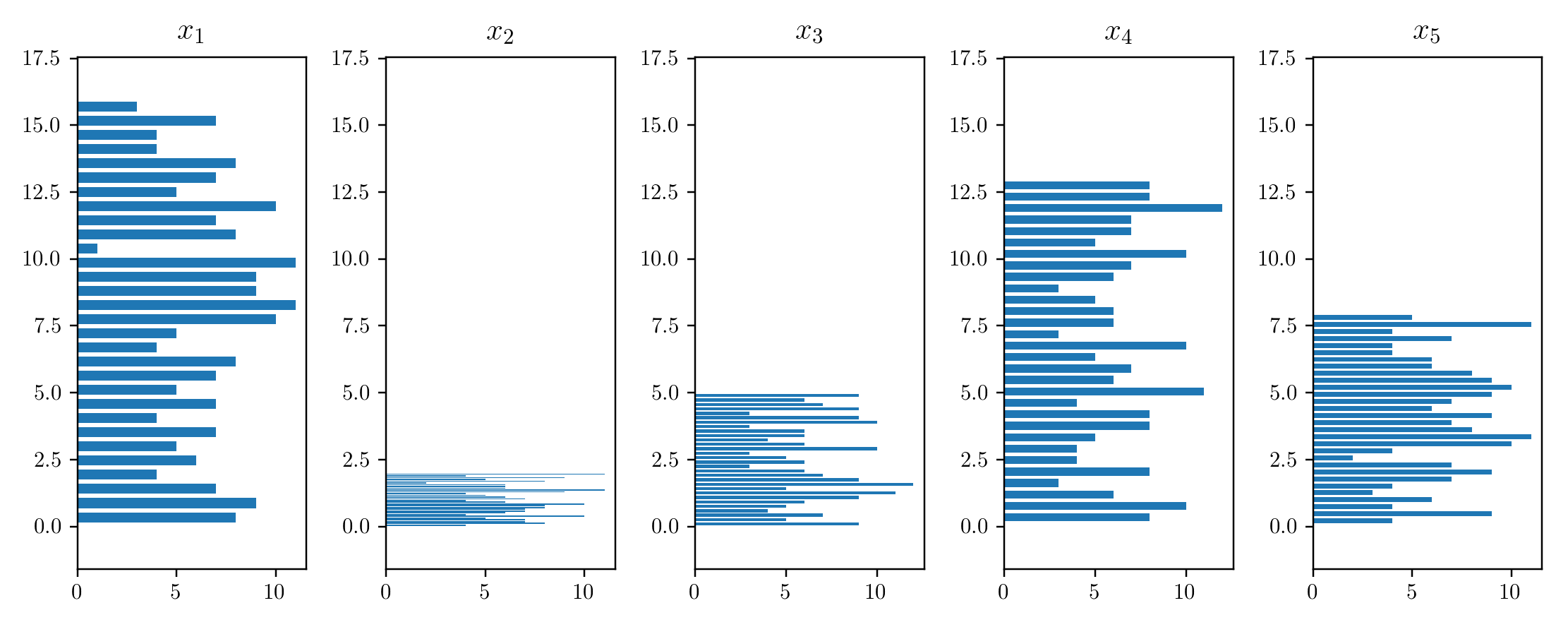As we can see in the plot above, the distributions of our input features here are way out of scale with each other, so we can expect gradient descent to converge quite slowly here - unless we normalize each input feature to have a similar distribution.

In analogy to the single-input case, here we should normalize each feature individually - that is each coordinate direction $x_n$. What are we trying to avoid by doing this? The (common) scenario where the distribution of input along each individual input dimensions widely varies, since this leads to a cost function with long narrow valley(s) that substantially slows down gradient descent.

Thus with the aim of standardizing each input direction - also referred to as a feature - we should normalize the $n^{th}$ input dimension of an $N$-input dimensional dataset $\left\{\mathbf{x}_p,y_p\right\}_{p=1}^P$ as

\begin{equation} x_{p,n} \longleftarrow \frac{x_{p,n} - \mu_n}{\sigma_n} \end{equation}

where $x_{p,n}$ is the $n^{th}$ coordinate of point $\mathbf{x}_p$ and $\mu_n$ and $\sigma_n$ are the mean and standard deviation of the $n^{th}$ dimension of the data, respectively, and are defined as

\begin{array} \ \mu_n = \frac{1}{P}\sum_{p=1}^{P}x_{p,n} \\ \sigma_n = \sqrt{\frac{1}{P}\sum_{p=1}^{P}\left(x_{p,n} - \mu_n \right)^2} \end{array}

Note that if $\sigma_n = 0$ for some $n$ the formula above makes little sense. However in this case the corresponding input feature is redundant and should be removed from the dataset entirely, since this implies that each and every $x_{p,n}$ is exactly the same constant value and thus absolutely nothing can be learned from its presence in the model. Indeed checking that the standard deviation of an input feature is greater than zero is a principle way of removing redundant input features from a dataset.

Now lets look at the distribution of our normalized input features.

In :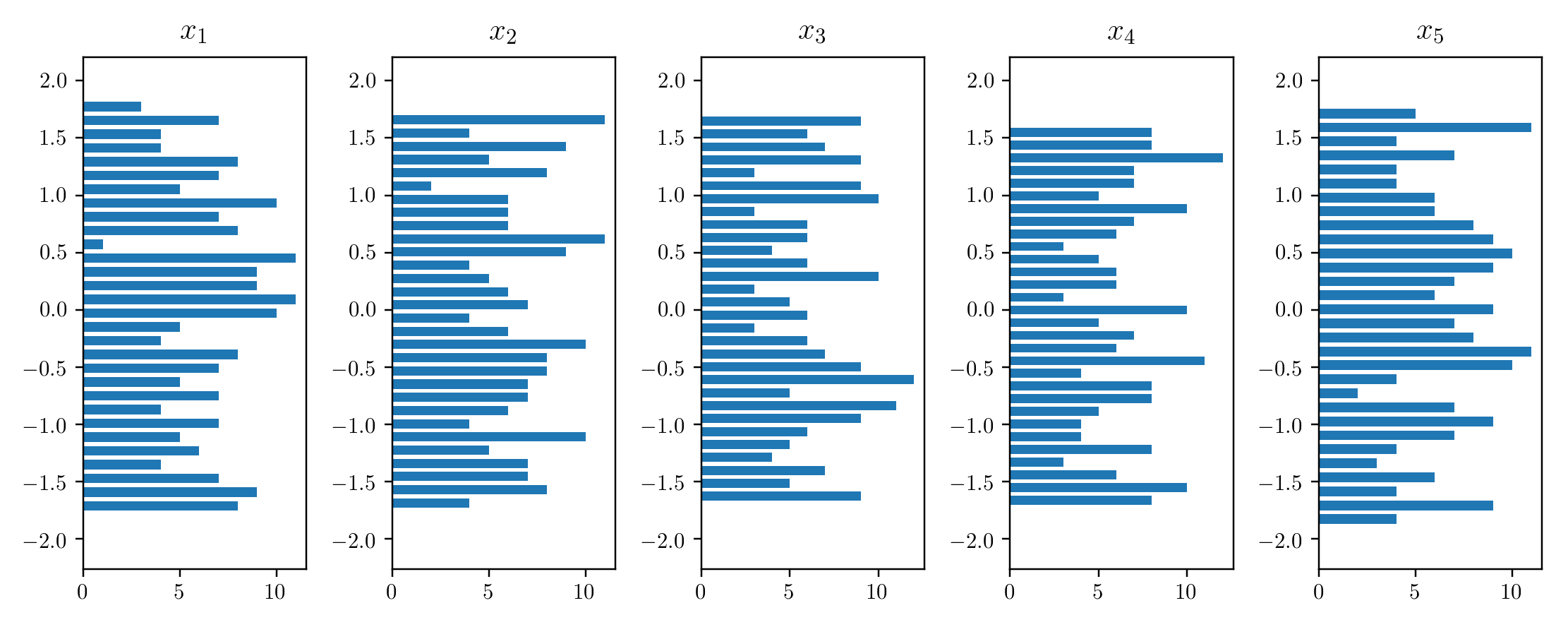Much better! With each input distribution normalized and roughly looking the same we can intuit, no individual weight $w_n$ will be significantly more sensitive (to proper tuning) than the others, and we can expect gradient descent to have a much easier time here.

Lets make a similar run of gradient descent using both the original and normalized data, comparing the results in terms of the cost function history plots. Here we use the same initial point, and the smallest fixed steplength parameter in each instance of the form $10^{-\gamma}$ for nonnegative $\gamma$ that produced convergence.

In :